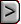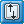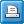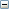﻿ SWI2 - Seawater Intrusion Package
Online Guide to MODFLOW-2005

SWI2 - Seawater Intrusion PackageSWI2 - Seawater Intrusion PackageSWI2 - Seawater Intrusion PackageAbbreviation in Name file

SWI2

Purpose

The SWI2 Package allows three-dimensional vertically integrated variable-density groundwater flow and seawater intrusion in coastal multiaquifer systems to be simulated using MODFLOW-2005.

Documentation

Bakker, M., Schaars, F., Hughes, J.D., Langevin, C.D., Dausman, A.M., 2013, Documentation of the seawater intrusion (SWI2) package for MODFLOW: U.S. Geological Survey Techniques and Methods, book 6, chap. A46, 47 p., https://pubs.usgs.gov/tm/6a46/.

Supported in

 • MODFLOW-2005
 • MODFLOW-NWT
 • MODFLOW-OWHM

Other Notes

The SWI2 package is not intended for the simulation of the combined flow of freshwater and brines, because variations in viscosity are neglected.

It is important to recognize that the steady-state head option does not mean that the steady-state density distribution is computed. The steady-state density distribution can only be computed by running the simulation until the density distribution is no longer changing.

Starting heads (STRT) should be modified to represent the freshwater head at the top of each layer.

All standard MODFLOW packages may be used with the SWI2 Package. When the package requires specification of a head, for example the General Head Boundary (GHB) Condition Package, this head must be the freshwater head at the top of the layer.

Similar to constant- and initial-head (STRT) values in the Basic (BAS) Package, time-variant specified head values for cells representing fluid densities greater than freshwater should be modified to represent the freshwater head at the top of each cell.

The SWI2 documentation contains tips for designing MODFLOW-2005 models using the SWI2 package.

Simulation of vertically integrated variable-density groundwater flow using the seawater intrusion (SWI2) Package is activated by including a record in the MODFLOW name file using the file type (Ftype) “SWI2” to indicate that relevant calculations are to be made in the model and to specify the related input data file. The NAM file should also be modified to include the appropriate information for saving interface (ZETA) elevations, SWI2 cell-by-cell budget data, and (or) SWI2 observation well data, if necessary.

The SWI2 file contains solver variables, data values for the different zones, algorithm parameters, the initial positions of the surfaces, the type of sources and sinks, and ZETA observation locations. In the body of the report, the number of surfaces are defined as being equal to the number of zones plus one. This number includes overlying and underlying surfaces corresponding to the layer top and bottom. In the input and output described here, however, only active ZETA surfaces are read and written. Active surfaces include only those surfaces between zones, and do not include the surfaces corresponding to the layer top and bottom. Thus, the number of active surfaces is equal to the number of zones minus one. Optional variables are indicated in [brackets].

FOR EACH SIMULATION

Data Set 0

[#Text]

Item 0 is optional—“#” must be in column 1. Item 0 can be repeated multiple times.Text
 Text—is a character variable (199 characters) that starts in column 2. Any characters can be included in Text. The “#” character must be in column 1. Except for the name file, lines beginning with # are restricted to these first lines of the file. Text is printed when the file is read.

Data Set 1

NSRF ISTRAT NOBS ISWIZT ISWIBD ISWIOBS [OPTIONS]Explanation of variablesNSRF
 Number of active surfaces (interfaces). This equals the number of zones minus one.ISTRAT

Flag indicating density distribution.

 • 0 – density varies linearly between surfaces.
 • 1 – density is constant between surfaces.NOBS
 Number of OBS observation locations.ISWIZT

Flag and a unit number for ZETA output.

 • If ISWIZT > 0, unit number for ZETA output
 • If ISWIZT ≤ 0, ZETA will not be recorded.

If ISWIZT > 0, ZETA output is written with the same frequency as HEAD output specified in the Output Control (OC) file.ISWIBD

Flag and a unit number for BUDGET output. When this option is selected, corrections to the cell by cell flows computed by MODFLOW will be written to the same or different file (depending on the unit number). Corrections are called SWIADDTOFLF, SWIADDTOFRF, and SWIADDTOFFF, for the lower face (LF), right face (RF) and front face (FF), respectively

 • If ISWIBD > 0, unit number for BUDGET
 • If ISWIBD ≤ 0, BUDGET will not be recorded.ISWIOBS

Flag and a unit number for OBS output

 • If ISWIOBS > 0, unit number for ASCII OBS
 • If ISWIOBS = 0, OBS will not be recorded.
 • If ISWIOBS < 0, |ISWIOBS| unit number for binary OBS.[OPTIONS]

Is an optional list of character values

 • “ADAPTIVE” —defines that adaptive SWI2 time step variables will be specified in dataset 3b.
 • "FSSSOPT" DEBUG OPTION - ADDITION DATA DURING TIP/TOE

Data Set 2a

NSOLVER IPRSOL MUTSOLExplanation of variablesNSOLVER

Flag indicating solver used to solve for ZETA surfaces

 • If NSOLVER = 1, the MODFLOW DE4 solver will be used.
 • If NSOLVER = 2, the MODFLOW PCG solver will be used. The PCG solver should be used for large problems when the time required for the DE4 solver to obtain a solution is excessive.IPRSOL
 is the printout interval for printing convergence information. If IPRSOL is equal to zero, it is changed to 999. The maximum ZETA change (positive or negative) and residual change are printed for each iteration of a time step whenever the time step is an even multiple of IPRSOL.MUTSOL

is a flag that controls printing of convergence information from the solver.

 • If MUTSOL = 0, tables of maximum head change and residual will be printed each iteration.
 • If MUTSOL = 1, only the total number of iterations will be printed.
 • If MUTSOL = 2, no information will be printed.
 • If MUTSOL = 3, information will only be printed if convergence fails.

IF NSOLVER = 2

Data Set 2b

MXITER ITER1 NPCOND ZCLOSE RCLOSE RELAX NBPOL DAMP [DAMPT]Explanation of variablesMXITER
 Maximum number of outer iterations—that is, calls to the solution routine.ITER1
 Maximum number of inner iterations—that is, iterations within the solution routine.NPCOND

flag used to select the matrix conditioning method for the MODFLOW PCG solver (NSOLVER = 2).

 • If NPCOND = 1, is for Modified Incomplete Cholesky (for use on scalar computers).
 • If NPCOND = 2, is for Polynomial (for use on vector computers or to conserve computer memory).ZCLOSE
 is the ZETA change criterion for convergence, in units of length. When the maximum absolute value of ZETA change from all nodes during an iteration is less than or equal to ZCLOSE, and the criterion for RCLOSE is also satisfied (see below), iteration stops.RCLOSE
 is the residual criterion for convergence, in units of cubic length per time. The units for length and time are the same as established for all model data. (See LENUNI and ITMUNI input variables in the Discretization File.) When the maximum absolute value of the residual at all nodes during an iteration is less than or equal to RCLOSE, and the criterion for ZCLOSE is also satisfied (see above), iteration stops.RELAX
 is the relaxation parameter used with NPCOND = 1. Usually, RELAX = 1.0, but for some problems a value of 0.99, 0.98, or 0.97 will reduce the number of iterations required for convergence. RELAX is only used if NSOLVER is 2 and NPCOND is 1.NBPOL
 is only specified when NSOLVER = 2 and used when NPCOND = 2 to indicate whether the estimate of the upper bound on the maximum eigenvalue is 2.0, or whether the estimate will be calculated. NBPOL = 2 is used to specify the value is 2.0; for any other value of NBPOL, the estimate is calculated. Convergence is generally insensitive to this parameter. NBPOL is not used if NPCOND is not 2.DAMP

is the damping factor and is only specified when NSOLVER = 2. It is typically set equal to one, which indicates no damping. A value less than 1.0 and greater than 0.0 causes damping.

 • If DAMP > 0, applies to both steady-state and transient stress periods.
 • If DAMP < 0, applies to steady-state periods. The absolute value if used as the dampening factor.[DAMPT]
 is the damping factor for transient stress periods and is only specified when NSOLVER = 2. DAMPT is enclosed in brackets indicating that it is an optional variable that only is read when DAMP is specified as a negative value. If DAMPT is not read, then the single damping factor, DAMP, is used for both transient and steady-state stress periods.

Data Set 3a

TOESLOPE TIPSLOPE [ALPHA] [BETA]Explanation of variablesTOESLOPE
 Maximum slope of toe cells.TIPSLOPE
 Maximum slope of tip cells.[ALPHA]
 Fraction of threshold used to move the tip and toe to adjacent empty cells when the slope exceeds user-specified TOESLOPE and TIPSLOPE values. If ALPHA is not specified, a default value of 0.1 is used. ALPHA must be greater than 0.0 and less than or equal to 1.0.[BETA]
 Fraction of threshold used to move the toe to adjacent non-empty cells when the surface is below a minimum value defined by the user-specified TOESLOPE value. A default BETA value of 0.1 is used if ALPHA is not specified. BETA must be greater than 0.0 and less than or equal to 1.0.

Data Set 3bExplanation of variablesNADPTMX
 Maximum number of SWI2 time steps per MODFLOW time step. If NADPTMX is less than 1, it is changed to 1.NADPTMN
 Minimum number of SWI2 time steps per MODFLOW time step. If NADPTMN is less than 1, it is changed to 1. NADPTMN must be less than or equal to NADPTMX.ADPTFCT
 is the factor used to evaluate tip and toe thicknesses and control the number of SWI2 time steps per MODFLOW time step. When the maximum tip or toe thickness exceeds the product of TOESLOPE or TIPSLOPE the cell size and ADPTFCT, the number of SWI2 time steps are increased to a value less than or equal to NADPT. When the maximum tip or toe thickness is less than the product of TOESLOPE or TIPSLOPE the cell size and ADPTFCT, the number of SWI2 time steps is decreased in the next MODFLOW time step to a value greater than or equal to 1. ADPTFCT must be greater than 0.0 and is reset to 1.0 if NADPTMX is equal to NADPTMN.

Data Set 4

NU(ISTRAT=0: NSRF+2, ISTRAT=1: NSRF+1)NU

Values of the dimensionless density

 • ISTRAT = 1 – Density of each zone (NSRF+1 values).
 • ISTRAT = 0 – Density along top of layer, each surface, and bottom of layer (NSRF+2 values)

FOR EACH SURFACE (FROM 1 to NSRF)

FOR EACH LAYER

Data Set 5

ZETA(NCOL,NROW) U2DRELZETA
 Initial elevations of the active surfaces.

FOR EACH LAYER

Data Set 6

SSZ(NCOL,NROW) U2DRELSSZ
 Effective porosity

FOR EACH LAYER

Data Set 7

ISOURCE(NCOL,NROW) U2DINTISOURCE

Source type of any external sources or sinks, specified with any outside package (i.e. WEL Package, RCH Package, GHB Package). There are three options:

 • If ISOURCE > 0 – Sources and sinks have the same fluid density as the zone ISOURCE. If such a zone is not present in the cell, sources and sinks have the same fluid density as the active zone at the top of the aquifer.
 • If ISOURCE = 0 – Sources and sinks have the same fluid density as the active zone at the top of the aquifer.
 • If ISOURCE < 0 – Sources have the same fluid density as the zone with a number equal to the absolute value of ISOURCE. Sinks have the same fluid density as the active zone at the top of the aquifer. This option is useful for the modeling of the ocean bottom where infiltrating water is salt, yet exfiltrating water is of the same type as the water at the top of the aquifer.

IF NOBS > 0

FOR EACH NOBS

Data Set 8

OBSNAM LAYER ROW COLUMNExplanation of variablesOBSNAM
 is a string of 1 to 12 nonblank characters used to identify the observation. The identifier need not be unique; however, identification of observations in the output files is facilitated if each observation is given a unique OBSNAM.LAYER
 is the layer index of the cell in which the ZETA observation is located.ROW
 is the row index of the cell in which the ZETA observation is located.COLUMN
 is the column index of the cell in which the ZETA observation is located.